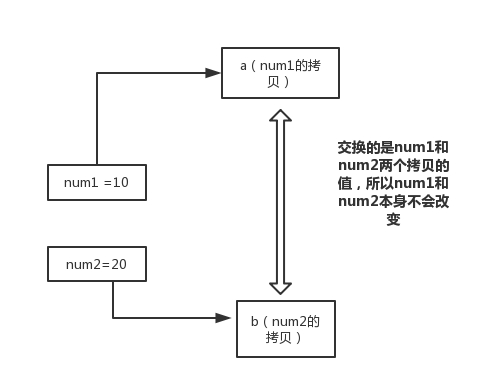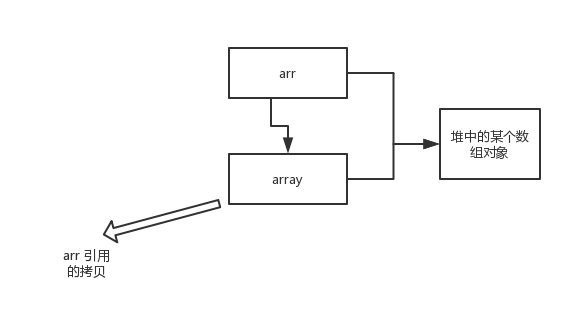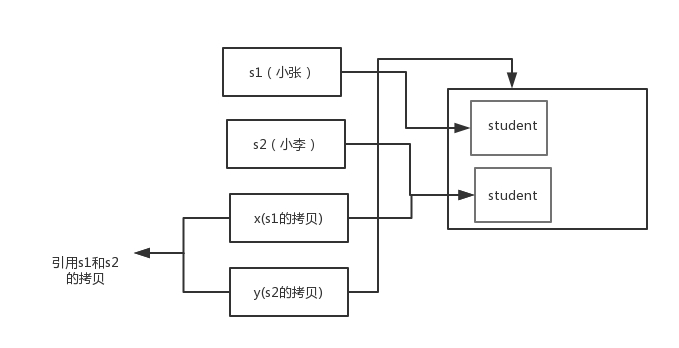# 为什么 Java 中只有值传递？【详细解释】

Java程序设计语言总是采用按值调用。也就是说，方法得到的是所有参数值的一个拷贝，也就是说，方法不能修改传递给它的任何参数变量的内容。

example 1

```

public static void main(String[] args) {
int num1 = 10;
int num2 = 20;

swap(num1, num2);

System.out.println("num1 = " + num1);
System.out.println("num2 = " + num2);
}

public static void swap(int a, int b) {
int temp = a;
a = b;
b = temp;

System.out.println("a = " + a);
System.out.println("b = " + b);
}

```

```
a = 20
b = 10
num1 = 10
num2 = 20

```example 2

```

public static void main(String[] args) {
int[] arr = { 1, 2, 3, 4, 5 };
System.out.println(arr);
change(arr);
System.out.println(arr);
}

public static void change(int[] array) {
// 将数组的第一个元素变为0
array = 0;
}

```

```

1
0

```array 被初始化 arr 的拷贝也就是一个对象的引用，也就是说 array 和 arr 指向的时同一个数组对象。 因此，外部对引用对象的改变会反映到所对应的对象上。

example 3

```

public class Test {

public static void main(String[] args) {
// TODO Auto-generated method stub
Student s1 = new Student("小张");
Student s2 = new Student("小李");
Test.swap(s1, s2);
System.out.println("s1:" + s1.getName());
System.out.println("s2:" + s2.getName());
}

public static void swap(Student x, Student y) {
Student temp = x;
x = y;
y = temp;
System.out.println("x:" + x.getName());
System.out.println("y:" + y.getName());
}
}

```

```

x:小李
y:小张
s1:小张
s2:小李

```Java程序设计语言对对象采用的不是引用调用，实际上，对象引用是按值传递的。

Java

## 当一个对象被当作参数传递到一个方法后，此方法可改变这个对象的属性，并可返回变化后的结果，那么这里到底是值传递还是引用传递？【面试题详解】

2020-11-26 17:36:46

Java

## 值传递和引用传递有什么区别？【面试题详解】

2020-11-26 17:41:33

'); })();